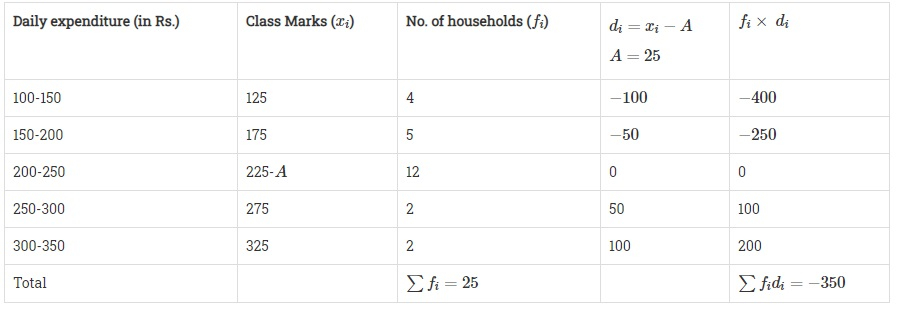# The table below shows the daily expenditure on food of 25 households in a locality.Daily expenditure ( in Rs ):100-150150-200200-250250-300300-350Number of households:451222Find the mean daily expenditure on food by a suitable method."

Given:

The daily expenditure on the food of 25 households in a locality.

To do:

We have to find the mean daily expenditure on food by a suitable method.

Solution:

Let the assumed mean be $A=225$.Mean $=A+\frac{\sum{f_id_i}}{\sum{f_i}}$

Therefore,

Mean $=225+(\frac{-350}{25})$

$=225-14$

$=211$

The mean daily expenditure on food is Rs. 211.